• matlab带通滤波器，可以很好的实现带通滤波。
• 应用切比雪夫滤波器，设计带通滤波器 function y=bandp(x,f1,f3,fsl,fsh,rp,rs,Fs) %带通滤波 %使用注意事项：通带或阻带的截止频率与采样率的选取范围是不能超过采样率的一半 %即，f1,f3,fs1,fsh,的值小于 Fs/2 %...
• matlab开发-带通滤波器设计使用gzTransforms。构造了中心频率为100Hz至>4G的两极双零带通滤波器。Simulink基础
• hbchifan的回答： % 用切比雪夫最佳一致逼近设计线性相位FIR带通滤波器； %信号为0.5hz, 0.9hz, 1.1hz和1.5hz的正统信号叠加组成 %通带为[0.9,1.1] %频谱分辨率与信号实际长度N成正比 clear all; f1=0.5;f2=0.9;f3=...
盘国英谭婷的回答：你自己整合吧，我没时间帮你整合，我给你提供一些程序：绝对正确的代码：程序1：fs=22050;%语音信号采样频率为22050x1=wavread('windowscriticalstop.wav');%读取语音信号的数据，赋给变量x1sound(x1,22050);%播放语音信号y1=fft(x1,1024);%对信号做1024点fft变换f=fs*(0:511)/1024;figure(1)plot(x1)%做原始语音信号的时域图形title('原始语音信号');xlabel('timen');ylabel('fuzhin');figure(2)freqz(x1)%绘制原始语音信号的频率响应图title('频率响应图')figure(3)subplot(2,1,1);plot(abs(y1(1:512)))%做原始语音信号的fft频谱图title('原始语音信号fft频谱')subplot(2,1,2);plot(f,abs(y1(1:512)));title('原始语音信号频谱')xlabel('hz');ylabel('fuzhi');程序2：fs=22050;%语音信号采样频率为22050x1=wavread('windowscriticalstop.wav');%读取语音信号的数据，赋给变量x1t=0:1/22050:(size(x1)-1)/22050;y1=fft(x1,1024);%对信号做1024点fft变换f=fs*(0:511)/1024;x2=randn(1,length(x1));%产生一与x长度一致的随机信号sound(x2,22050);figure(1)plot(x2)%做原始语音信号的时域图形title('高斯随机噪声');xlabel('timen');ylabel('fuzhin');randn('state',0);m=randn(size(x1));x2=0.1*m+x1;sound(x2,22050);%播放加噪声后的语音信号y2=fft(x2,1024);figure(2)plot(t,x2)title('加噪后的语音信号');xlabel('timen');ylabel('fuzhin');figure(3)subplot(2,1,1);plot(f,abs(y2(1:512)));title('原始语音信号频谱');xlabel('hz');ylabel('fuzhi');subplot(2,1,2);plot(f,abs(y2(1:512)));title('加噪后的语音信号频谱');xlabel('hz');ylabel('fuzhi');根据以上代码，你可以修改下面有错误的代码程序3:双线性变换法设计butterworth滤波器fs=22050;x1=wavread('h:\课程设计2\shuzi.wav');t=0:1/22050:(size(x1)-1)/22050;au=0.03;d=[au*cos(2*pi*5000*t)]';x2=x1+d;wp=0.25*pi;ws=0.3*pi;rp=1;rs=15;fs=22050;ts=1/fs;wp1=2/ts*tan(wp/2);%将模拟指标转换成数字指标ws1=2/ts*tan(ws/2);[n,wn]=buttord(wp1,ws1,rp,rs,'s');%选择滤波器的最小阶数[z,p,k]=buttap(n);%创建butterworth模拟滤波器[bap,aap]=zp2tf(z,p,k);[b,a]=lp2lp(bap,aap,wn);[bz,az]=bilinear(b,a,fs);%用双线性变换法实现模拟滤波器到数字滤波器的转换[h,w]=freqz(bz,az);%绘制频率响应曲线figure(1)plot(w*fs/(2*pi),abs(h))gridxlabel('频率／hz')ylabel('频率响应幅度')title('butterworth')f1=filter(bz,az,x2);figure(2)subplot(2,1,1)plot(t,x2)%画出滤波前的时域图title('滤波前的时域波形');subplot(2,1,2)plot(t,f1);%画出滤波后的时域图title('滤波后的时域波形');sound(f1,22050);%播放滤波后的信号f0=fft(f1,1024);f=fs*(0:511)/1024;figure(3)y2=fft(x2,1024);subplot(2,1,1);plot(f,abs(y2(1:512)));%画出滤波前的频谱图title('滤波前的频谱')xlabel('hz');ylabel('fuzhi');subplot(2,1,2)f1=plot(f,abs(f0(1:512)));%画出滤波后的频谱图title('滤波后的频谱')xlabel('hz');ylabel('fuzhi');程序4:窗函数法设计滤波器：fs=22050;x1=wavread('h:\课程设计2\shuzi.wav');t=0:1/22050:(size(x1)-1)/22050;au=0.03;d=[au*cos(2*pi*5000*t)]';x2=x1+d;wp=0.25*pi;ws=0.3*pi;wdelta=ws-wp;n=ceil(6.6*pi/wdelta);%取整wn=(0.2+0.3)*pi/2;b=fir1(n,wn/pi,hamming(n+1));%选择窗函数，并归一化截止频率figure(1)freqz(b,1,512)f2=filter(bz,az,x2)figure(2)subplot(2,1,1)plot(t,x2)title('滤波前的时域波形');subplot(2,1,2)plot(t,f2);title('滤波后的时域波形');sound(f2,22050);%播放滤波后的语音信号f0=fft(f2,1024);f=fs*(0:511)/1024;figure(3)y2=fft(x2,1024);subplot(2,1,1);plot(f,abs(y2(1:512)));title('滤波前的频谱')xlabel('hz');ylabel('fuzhi');subplot(2,1,2)f2=plot(f,abs(f0(1:512)));title('滤波后的频谱')xlabel('hz');ylabel('fuzhi');hbchifan的回答：% 用切比雪夫最佳一致逼近设计线性相位FIR带通滤波器；%信号为0.5hz, 0.9hz, 1.1hz和1.5hz的正统信号叠加组成%通带为[0.9,1.1]%频谱分辨率与信号实际长度N成正比clear all;f1=0.5;f2=0.9;f3=1.1;f4=1.5;t=0:1203;N=length(t);fs=10;M=512;x1=sin(2*pi*(f1/fs)*t)+sin(2*pi*(f2/fs)*t)+sin(2*pi*(f3/fs)*t)+sin(2*pi*(f4/fs)*t);figure(1);subplot(211);plot(t,x1);title('原信号');y=fft(x1);f=(0:1/N:1/2-1/N)*fs;subplot(212);plot(f,abs(y(1:N/2)));grid;xlabel('hz');%处理前频谱wc1=2*f2/fs;wc2=2*f3/fs;wc3=2*f4/fs;%归一化角频率，用于下面的f1f1=[0 wc1-0.05 wc1 wc2 wc2+0.05 1];A=[0 0 1 1 0 0];%设置带通或带阻，1为带通，0为带阻weigh=[1 1 1 ];%设置通带和阻带的权重b=remez(60,f1,A,weigh);%传函分子h1=freqz(b,1,M);%幅频特性figure(2)f=(0:1/M:1-1/M)*fs/2;subplot(211);plot(f,abs(h1));grid;title('带通');x2=filter(b,1,x1);S1=fft(x2);f=(0:1/N:1/2-1/N)*fs;subplot(212);plot(f,abs(S1(1:N/2)));grid;xlabel('hz');%处理后频谱
展开全文• 预备知识 关于电容HPF 的推导 simulink 仿真simulink 运行结果matlab 实现matlab 运行结果C 语言实现预备知识高通滤波器(HPF-high pass filter)可以滤除频率低于截止频率的信号，类似的还有低通滤波器，带通滤波器，...
预备知识 关于电容HPF 的推导simulink 仿真simulink 运行结果matlab 实现matlab 运行结果C 语言实现预备知识高通滤波器(HPF-high pass filter)可以滤除频率低于截止频率的信号，类似的还有低通滤波器，带通滤波器，带阻滤波器。一阶 RC 高通滤波器的电路如下图所示；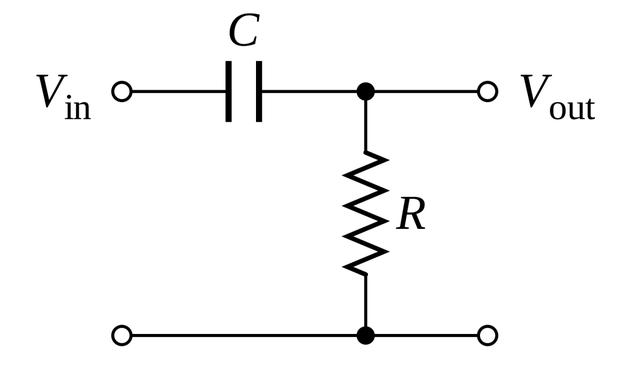关于电容首先对电容的几个公式做一下补充；电容大小满足；其中 是电容所带的电荷量， 是电容两端的电势差；另外，电流相当于单位时间流过导体的电荷量；因此电流 满足；根据①，②可以得到电容大小 和电容的电流 以及两端电压 的关系；HPF 的推导由以上电路可知，假设电流为 i(t) ，则可知电容两端的电压为 Vc(t) 根据基尔霍夫定律，满足；所以结合①，③，④可以得到；根据 ③，④，⑤ 可以得到以下关系；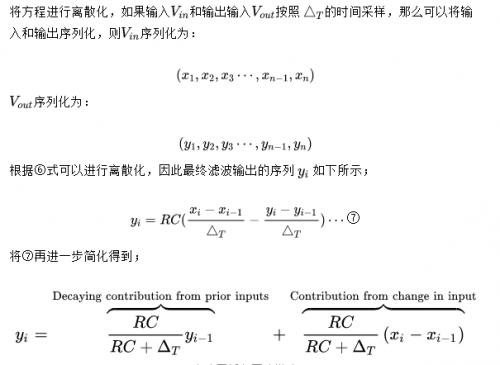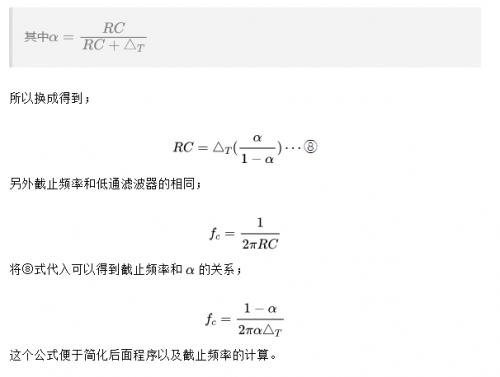simulink 仿真这里根据公式⑥构建simulink的子模块subsystem；具体如下所示；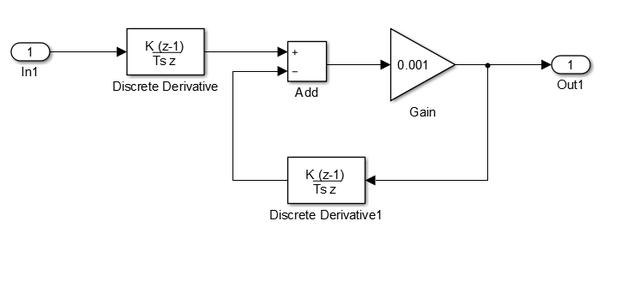整体的仿真如下图所示；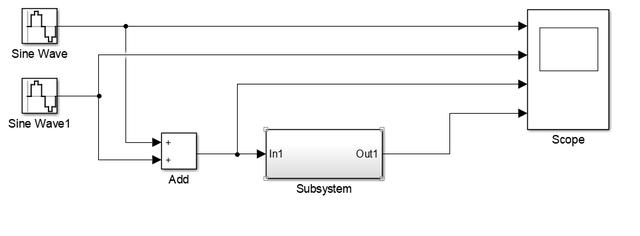其中Sine Wave频率设置为2*pi*40，频率为40赫兹;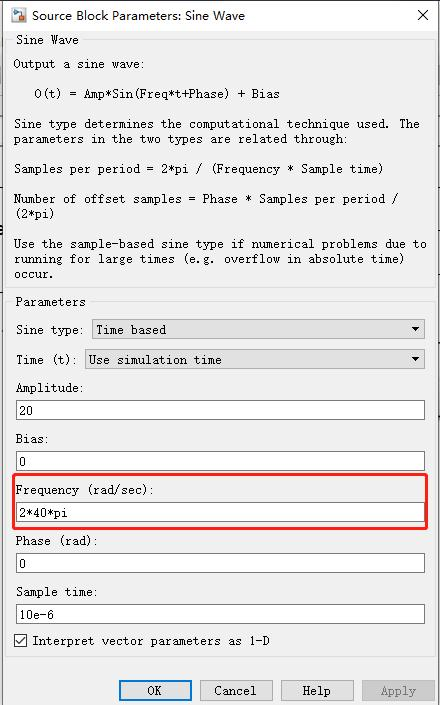其中Sine Wave1频率设置为 2*pi*4，频率为4赫兹;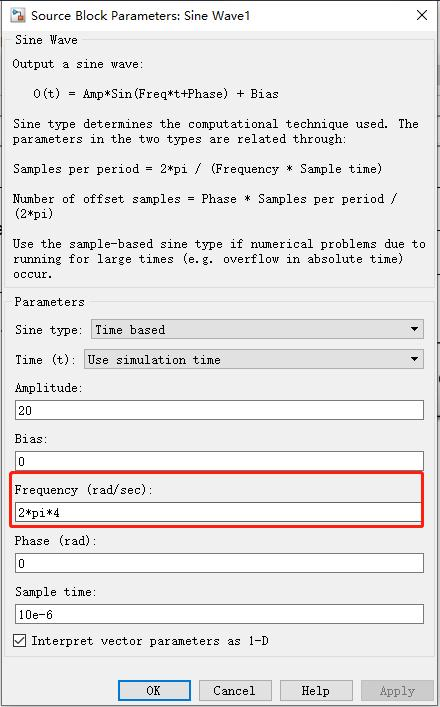所以这里需要使得2*pi*4的信号衰减，所以根据，截止频率 fc的计算公式，可以改变增益的值，具体如下所示；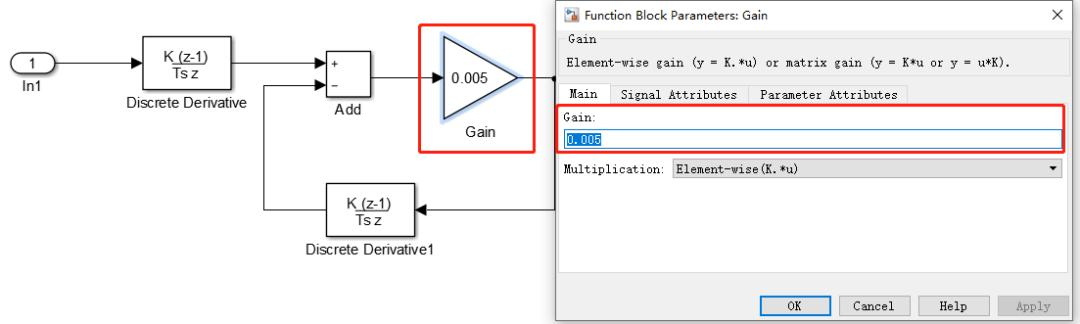这里RC增益为0.005，因此simulink 运行结果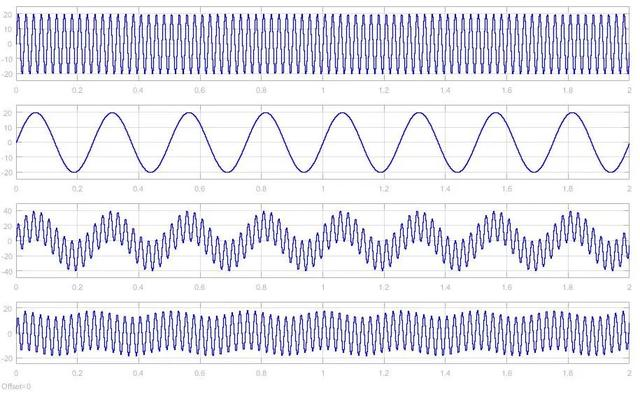matlab 实现matlab根据以下这个公式进行数字滤波器的设计；另外 的值如何确定需要参考⑧式；Serial = 0:0.1:100;Fs = 1;Phase = 0;Amp = 1;N0 = 2*pi*Fs*Serial - Phase;X0 = Amp*sin(N0);subplot(4,1,1);plot(X0);Fs = 0.02;N1 = 2*pi*Fs*Serial - Phase;X1 = Amp*sin(N1);subplot(4,1,2);plot(X1);X2=X0+X1;subplot(4,1,3);plot(X2);len = length(X2);X3=X2;p=0.75;for i=2:len    X3(i) = p*X3(i-1)+p*(X2(i)- X2(i-1))endsubplot(4,1,4);plot(X3);简单地分析一下，代码中的X1，X2，X3；X1 频率为1X2 频率为0.02因此可以得到截止频率如下；matlab 运行结果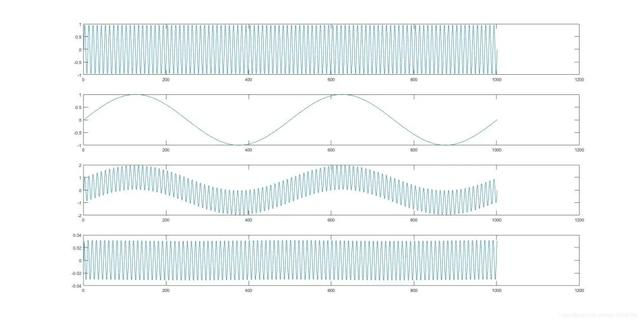C 语言实现typedef struct{     int16_t  Input;     int16_t  Output;     int32_t  FilterTf;       int32_t  FilterTs;     int32_t  Ky;} high_filter;void high_filter_init(high_filter *v);int16_t high_filter_calc(high_filter *v);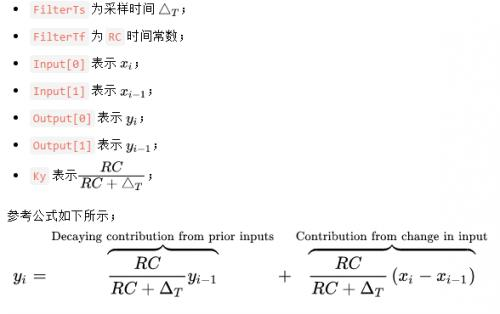void high_filter_init(high_filter *v){      v->Ky = v->FilterTf*1024/(v->FilterTs + v->FilterTf);}int16_t high_filter_calc(high_filter *v){ int32_t tmp = 0; tmp = ((int32_t)v->Ky*v->Output + v->Ky*(v->Input - v->Input))/1024; if(tmp>32767){  tmp = 32767; }  if( tmp < -32768){  tmp = -32768; }     v->Output = (int16_t)tmp;    v->Output = v->Output;    v->Input = v->Input; return v->Output;}
展开全文• 基于Matlab的FIR带通滤波器设计及DSP实现.pdf
• 0 引言近年来，随着移动通信系统、雷达系统以及超宽带通信系统的发展，小型化、宽阻带性能的滤波器在实际应用中受到了广泛关注。传统的并联分支线低通滤波器和半波长平行耦合线滤波器的寄生通带都位于中心频率的2倍...
0 引言近年来，随着移动通信系统、雷达系统以及超宽带通信系统的发展，小型化、宽阻带性能的滤波器在实际应用中受到了广泛关注。传统的并联分支线低通滤波器和半波长平行耦合线滤波器的寄生通带都位于中心频率的2倍处，而传统的阶跃阻抗谐振滤波器的寄生通带在中心频率的2.5倍处左右，应用时很难获得宽阻带的抑制效果。而且此类滤波器的尺寸较大，阻带窄，受微带加工最小宽度的限制，滤波器的性能受到一定的制约。为了得到陡峭的衰减边沿及更好的阻带特性，需要增加短路或开路短截线数，但这会进一步增大电路尺寸，并且在通带内引入更多的插入损耗。通过在有限频率处引入传输零点可以获得较好的频率选择特性及带外抑制。在滤波器的设计中，交叉耦合被广泛用来在阻带引入有限传输零点，这些传输零点可以很好地改善带边过渡特性及阻带抑制能力。本文首先分析了阶跃阻抗谐振器的结构原理、三阶交叉耦合结构原理，随后提出了一种小型化宽阻带微带带通滤波器的设计方案，其寄生通带在中心频率的约4倍处，比一般的滤波器具有更宽的阻带，并对仿真与实测结果进行了分析，且得到了较好的一致性。1 基本设计理论1.1 阶跃阻抗谐振器原理阶跃阻抗谐振器常采用λg /4 型、λg/ 2 型或λg 型三种基本谐振结构，其中λg /2 型谐振器的基本结构如图1所示，为非等电长度半波长结构，由特征阻抗分别由Z1 和Z2 的传输线组成，其对应电长度为θ1 和θ2.如果忽略结构中的阶跃非连续性和开路端的边缘电容，从开路端看的输入导纳Yin 为：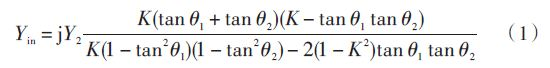式中：K 为阻抗比，定义为K = Z2 Z1.为设计方便，取θ1 = θ2 = θ，则式(1)简化为：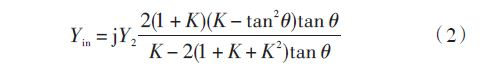其谐振条件为：Yin = 0，得其基频振荡条件为K = Z2 Z1 = tan 2θ。由此公式可知，阶跃阻抗谐振器的谐振条件取决于电长度θ 和阻抗比率K.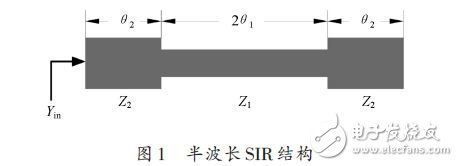1.2 三阶交叉耦合结构原理对于窄带滤波器，其三阶交叉耦合滤波器的等效电路如图2 所示。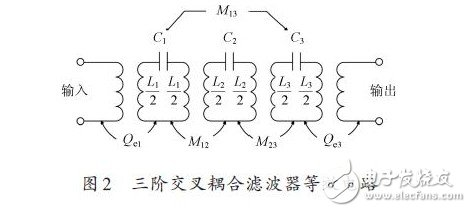相邻谐振器间的耦合用M12 和M23 表示，交叉耦合用M13 表示。外部品质因数Qe1 和Qe3 各表示输入和输出耦合。图2所示的耦合滤波器等效电路可以被转换为一个低通原型滤波器形式，如图3所示。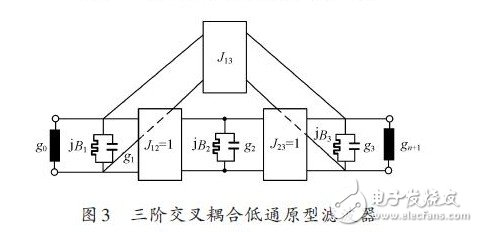其中每个矩形框代表一个频率不变的J 导纳变换器。在一个对称的二端口电路中，J12 = J23 = 1，g0 = g4 = 1，g1 = g3，B1 = B3.
展开全文• 用基本数学方程设计带通滤波器的程序，对没有信号处理工具箱的人有帮助。 三角正弦函数和汉明窗方程用于创建带通滤波器。 通过改变中心频率、带宽参数，滤波器可以定制到所需的频率。 可以参考相同的程序来创建 ...
• 基于matlab窗函数的FIR带通滤波器设计.pdf
• FIR滤波器设计文献集-基于Matlab的FIR带通滤波器的设计与仿真.pdf 本帖最后由 zyzhang 于 2012-4-24 18:52 编辑 载自各大数据库希望能帮到大家 基于Matlab的FIR带通滤波器的设计与仿真.pdf 基于...
• matlab设计FIR 带通滤波器，实例讲解，程序代码，图像均包含。
• 看到一个带通滤波器设计如下，开始研究是如何设计的 代码： band_low = 0.3; band_high = 1.2; fs_origin = 25; hd_band = design(fdesign.bandpass('N,F3dB1,F3dB2',4,band_low,band_high,fs_origin),'...
写在前面的话：

由滤波器系数得到传输函数：

使用matlab fdatool设计滤波器，得到幅频响应，得到滤波器系数，由系数如何得到滤波器的参数？

看到一个带通滤波器的设计如下，开始研究是如何设计的

带通滤波器设计matlab代码：

band_low = 0.3;
band_high = 1.2;
fs_origin = 25;
hd_band = design(fdesign.bandpass('N,F3dB1,F3dB2',4,band_low,band_high,fs_origin),'butter'); %带通滤波器
%fvtool(hd_band); % view
measure(hd_band) %Measure the frequency response characteristics of the filter
%filtered = filter(hd_band,x);  % 滤波器的使用，输入数组x,输出数组filtered

查看官网MathWorks,找到关于滤波器的设计design,带通滤波器的设计fdesign.bandpass

The fdesign.bandpass function returns a bandpass filter design specification object that contains specifications for a filter such as passband frequency, stopband frequency, passband ripple, and filter order. Use the design function to design the filter from the filter design specifications object.

本处只解析此处滤波器的设计参数，若查看更多，移至官网MathWorks

N:Filter order for FIR filters. Or both the numerator and denominator orders for IIR filters when Na and Nb are not provided. Specified using FilterOrder. 关于滤波其的阶数
F3dB1:Frequency of the 3 dB point below the passband value for the first cutoff, specified in normalized frequency units. Applies to IIR filters.
F3dB2:Frequency of the 3 dB point below the passband value for the second cutoff, specified in normalized frequency units. Applies to IIR filters.
Fs bandpassSpecs = fdesign.bandpass(___,Fs) provides the sample rate in Hz of the signal to be filtered. Fs must be specified as a scalar trailing the other numerical values provided. In this case, all frequencies in the specifications are in Hz as well.
上面这句话解释了传入采样频率的必要性。

'N,F3dB1,F3dB2'
butter
以上参数的设置使用与IIR butter

fdesign.bandpass('N,F3dB1,F3dB2',4,band_low,band_high,fs_origin)
show:
bandpass (具有属性):

Response: 'Bandpass'
Specification: 'N,F3dB1,F3dB2'
Description: {3×1 cell}
NormalizedFrequency: 0
Fs: 25
FilterOrder: 4
F3dB1: 0.3000
F3dB2: 1.2000

fvtool(hd_band)% 查看幅频特性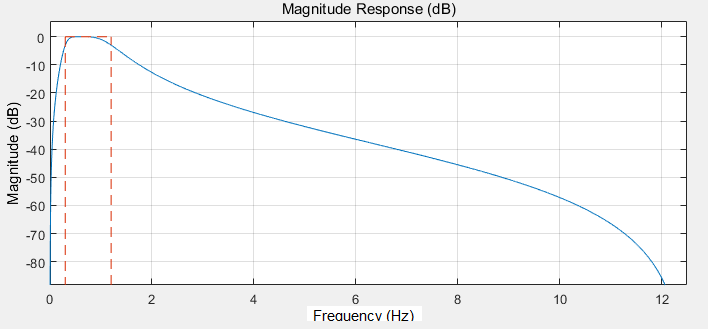measure(hd_band) %Measure the frequency response characteristics of the filter

Sample Rate             : 25 Hz
First Stopband Edge     : Unknown
First 6-dB Point        : 250.7041 mHz
First 3-dB Point        : 300 mHz
First Passband Edge     : Unknown
Second Passband Edge    : Unknown
Second 3-dB Point       : 1.2 Hz
Second 6-dB Point       : 1.4315 Hz
Second Stopband Edge    : Unknown
First Stopband Atten.   : Unknown
Passband Ripple         : Unknown
Second Stopband Atten.  : Unknown
First Transition Width  : Unknown
Second Transition Width : Unknown  

使用fdatool同样实现此滤波器的设计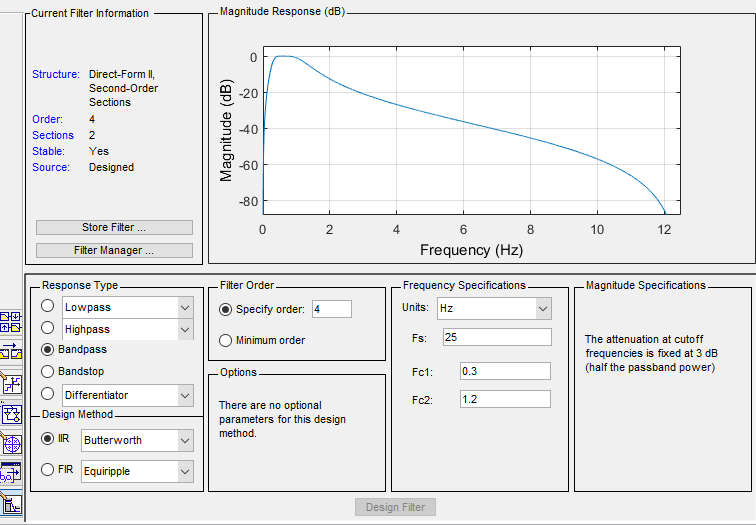设计的滤波器通过File-Export导出到工作区；

也可以在analysis中查看器信息，比如系数、幅频、相频特性。比如查看analysis中点击Filter Information中可以看到fdesign使用的内容：'N,F3dB1,F3dB2'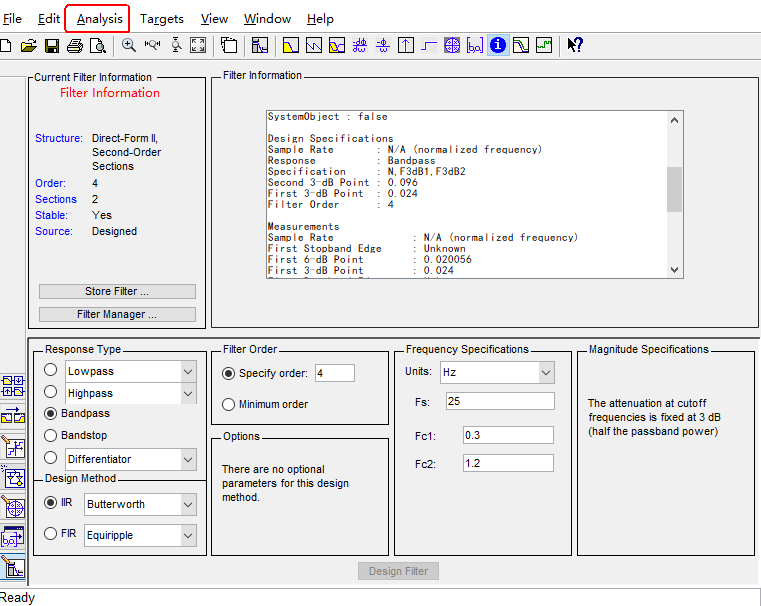完成了带通滤波器的设计，就在考虑如何转化为C语言代码，这里有个网站可以进行转化，设计滤波器转为C语言实现，但是结果并没有达到我想要的要求，我的目的是怎样将fdatool中设计的滤波器系数中得到其对应的传递函数H(Z)，有了传递函数，就可以自己写C语言实现了。

在上面fdatool设计的滤波器中，两种方法查看设计的滤波器，任意一种都可以；

或者采用如下图操作，从左到右三个按键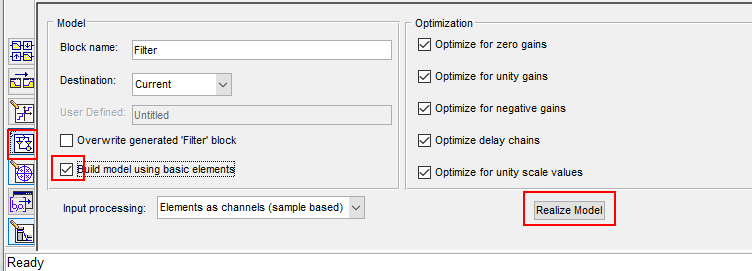双击可以看到滤波器的内部结构，如图显示为两个二阶滤波器级联：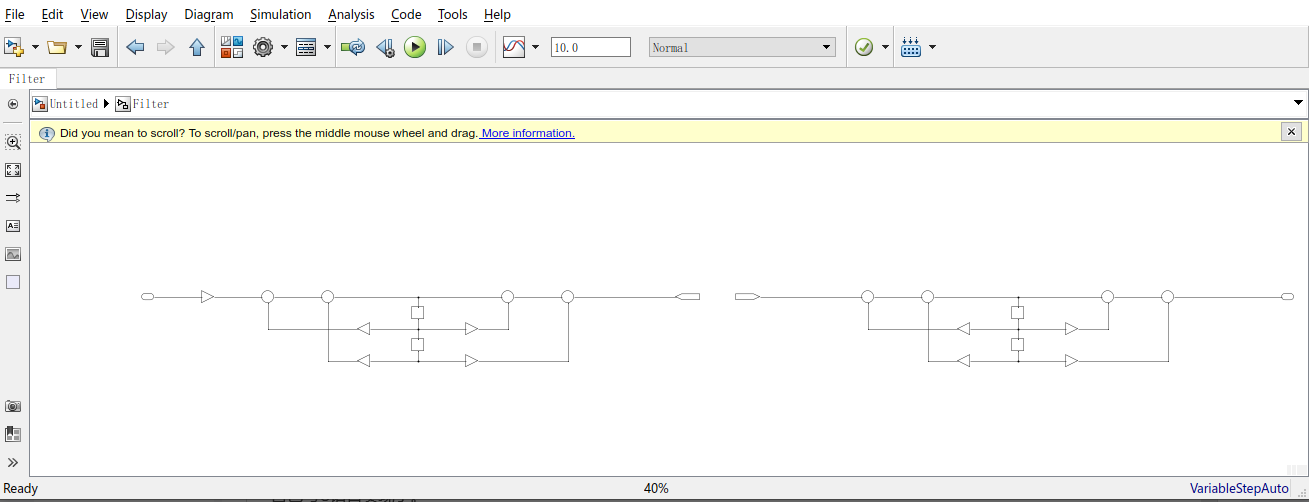放大其中一个滤波器如下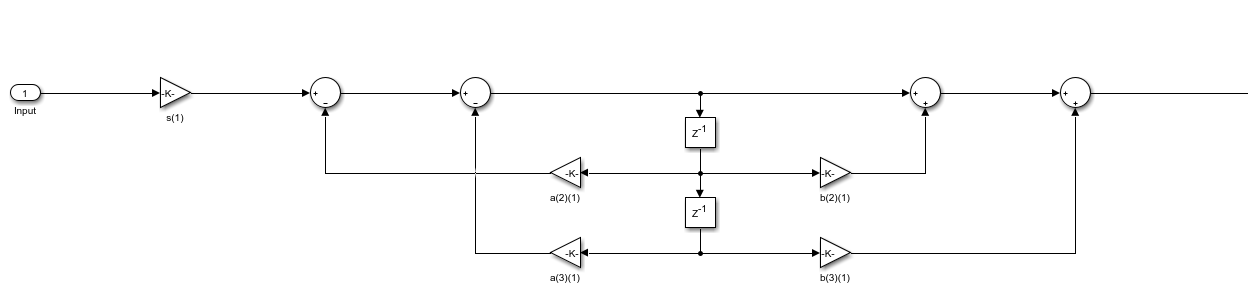简单作了标记如下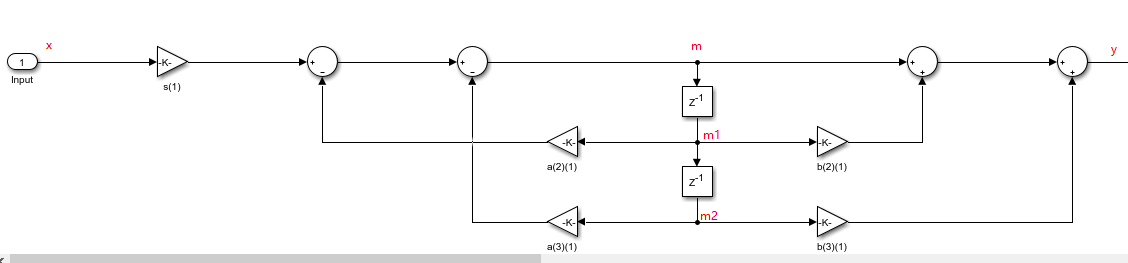并给出其对应的C语言实现，此代码无法直接运行只是提取了一部分带通滤波器实现部分

/* IIR fourth-order filter coefficients */
typedef struct
{
float(*a);
float(*b);
float* gain;
} IIR_ORDER4_COEFF_T;

/* Save the in-process' data of the second-order filter */
typedef struct
{
float m1;
float m2;
} FILTER_ORDER2_T;

/*三轴信号处理前的滤波：四阶带通(0.3-1.2)Hz)滤波，由两个二阶滤波级联起来*/
float a_raw = {
{ 1.00, (float)-1.9175958145710057, (float)0.9246554650412394 },    //第一级二阶滤波，分母
{ 1.00, (float)-1.7227109782351659, (float)0.7854234338937474 }    //第二级二阶滤波，分母
};//分母
float b_raw = {
{ 1.00, (float)0.00, (float)-1.00 },    //第一级二阶滤波，分子
{ 1.00, (float)0.00, (float)-1.00 }    //第二级二阶滤波，分子
};//分子
float gain_raw = { (float)0.1048485643154691/*第一级增益*/, (float)0.1048485643154691/*第二级增益*/ };

/******************************************************************************/
/** Start, initialize the parameters
* @param[in]
*
* @return
*
*//*
* History        :
* 1.Date         : 2020/8/28
*   Author       :
*   Modification : Created function

*******************************************************************************/
void StartSwim(void)
{
/* signal filter parameter initialization */
IIR_Raw.a = a_raw;
IIR_Raw.b = b_raw;
IIR_Raw.gain = gain_raw;
}

/*
x: input
m1:保存中间滤波器数据
m2:保存中间滤波器数据
a:滤波器系数
b:滤波器系数
gain:增益，对于只支持定点小数运算的，需要把增益分配到每个二阶IIR滤波器的系数中，使得每次中间的结果都不溢出，即使其频率响应的最大值最接近0dB
*/
float filterOrder2(float* x, float* m_1, float* m_2, float* a, float* b, float* gain){
float y, m;
//计算没有增益的滤波输出，存于y_
m = *x - a * *m_1 - a * *m_2;    //求当前m，同时作为求y_的中间步骤
y = m + b * *m_1 + b * *m_2;
//更新*m_1和*m_2
*m_2 = *m_1;
*m_1 = m;
//返回带增益的滤波输出
return y* *gain;
}

/******************************************************************************/
/** filter: Fourth-order filter, direct type II
* @param[in]
* filter_state	:Store the middle state of the filter
* @return
*
*//*
* History        :
* 1.Date         : 2020/8/28
*   Author       :
*   Modification : Created function

*******************************************************************************/
float FilterOrder4(float data, FILTER_ORDER4_T* filter_state, IIR_ORDER4_COEFF_T* IIR_coeffs)
{
float data_filtered;

filter_state->first_order2_out = filterOrder2(&data, &filter_state->first_order2.m1, &filter_state->first_order2.m2, IIR_coeffs->a, IIR_coeffs->b, IIR_coeffs->gain);  //第一级二阶滤波
data_filtered = filterOrder2(&filter_state->first_order2_out, &filter_state->second_order2.m1, &filter_state->second_order2.m2, IIR_coeffs->a, IIR_coeffs->b, &IIR_coeffs->gain);  //第二级二阶滤波

return data_filtered;
}

/******************************************************************************/
/** Band-pass filtering for the norm data: 0.25-2Hz
* @param[in] x:norm data
*
* @return filtered data
*
*//*
* History        :
* 1.Date         : 2020/8/28
*   Author       :
*   Modification : Created function

*******************************************************************************/
float BandFilter(float x)
{
float y = FilterOrder4(x, &xyz_filter, &IIR_vec);

return y;
}

关于fdatool设计的带通滤波器的系数使用

使用Matlab的FDAtool工具可以很方便地设计IIR滤波器。使用File菜单中的export选项可以把滤波器的参数输出到Matlab的工作空间中。若滤波器为IIR型，则输出的变量名为G和SOS。它把高阶的IIR滤波器转换为一系列二阶IIR滤波器的级联。SOS为二阶IIR滤波器的系数（b,a），G为各级的增益系数，可用来调节各级通带的衰减。计算IIR滤波器的输出的时候，先将输入数据乘以G, 然后一一通过SOS中的每个IIR滤波器，就得到最终的结果了。

SOS的每一行表示一级二阶IIR滤波器系[b0,b1,b2,a0,a1,a2],G的每个元素表示相应级的增益系数。也即将G乘上SOS的b0,b1,b2。得到一组新的滤波器系数SOS'，可调用MATLAB滤波函数sosfilt对输入信号进行滤波。

但是如果二阶IIR滤波器的程序只支持定点小数运算的话，那么就需要调整一下系数了。因为设计出来的G有时候很小（不知道会不会很大），这样一旦把输入数据乘以G后，就会使得二阶IIR滤波器的输入过小，造成很大的误差。我们需要把G分配到每个二阶IIR滤波器的系数中，使得每次得到的中间结果都刚好不会溢出。若以IIRi表示第i个二阶IIR滤波器的话，则我们需要保证IIR1, IIR1 * IIR2, IIR1 * IIR2 * IIR3, … 的频率响应都不超过0dB，*号表示级联。为了使得输出的精度最好，我们还需要让上面这些滤波器的频率响应中的最大值最接近0dB。

级联型：将系统传递函数H(z)因式分解为多个二阶子系统，系统函数就可以表示为这些二阶子系统传递函数的乘积。实现时将每个二阶子系统用直接型实现，整个系统函数用二阶环节的级联实现。

高阶IIR滤波器的实现是采用二阶滤波器级联的方式来实现的。默认情况下，Filter Coefficients把结果分成多个2阶Section显示，其中还有增益。增益的目的是为了保证计算的精度和系统的稳定性。

简单介绍低通滤波器的使用，其函数参考fdesign官网

如下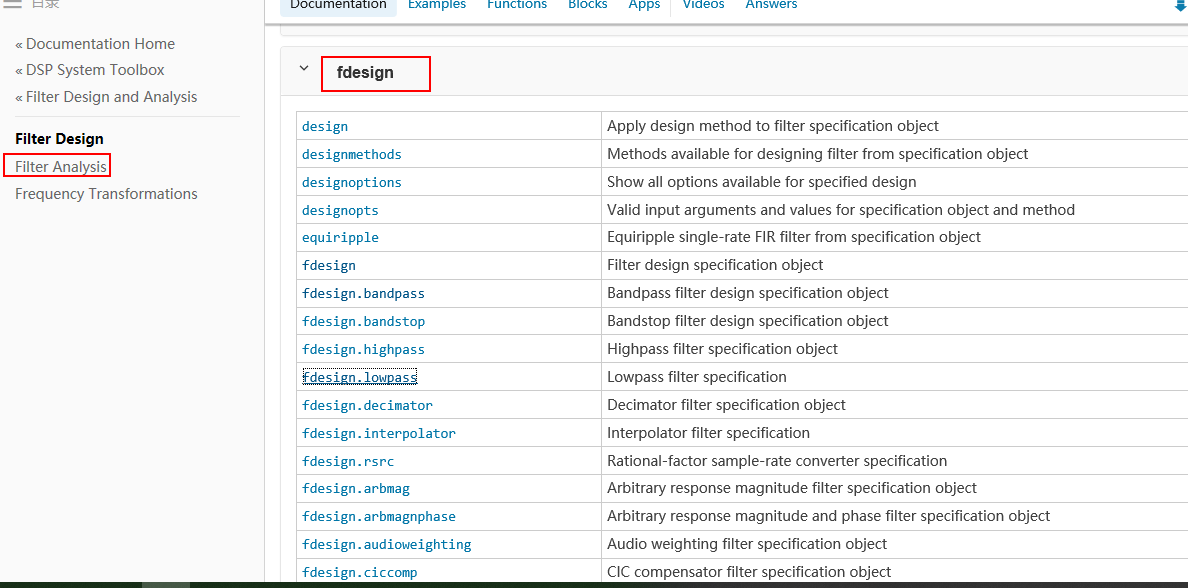简单设计了低通滤波

%% 低通滤波
function filtered = LowFilter(x)
fs_origin=1;
fc=0.03;
N=4;
hd_low = design(fdesign.lowpass('N,F3dB',N,fc, fs_origin),'butter');
filtered = filter(hd_low,x);
end

..


展开全文• 摘 要：本文以70MHz带通滤波器为例，论述了如何采用BESSEL函数进行精确带通滤波器设计，同时利用PSPICE和MATLAB软件对设计结果进行波特图和群延迟特性仿真。关键词：BESSEL函数；带通滤波器；PSPICE；MATLAB；群延迟...
• matlab汉宁窗的带通滤波器设计，希望有用
• 　模拟带通滤波器在信号的检测和传输过程中起着很重要的作用，但在传统模拟带通滤波器的设计过程中需要大量繁琐的数值计算，如果手工计算则费时费力。也有许多公司开发了相关的滤波器设计软件，如AnsoftDesigner，...
• 在前一段时间遇到了一个MATLAB带通滤波方面的问题，滤波后首尾段数据都出现异常状况，然后就开始疯狂的查资料，但是这方面的资料说实话确实很少，尤其是这种滤波器性能导致的问题，后来偶然的巧合下，在MATLAB中文...异常处理
• 基于Matlab带通滤波器综合分析软件.pdf
• ## matlab设计模拟带通滤波器

万次阅读 多人点赞 2018-10-24 21:11:13
简单记录下在matlab上如何设计出模拟的带通滤波器，包括：巴特沃斯滤波器、切比雪夫I型滤波器、切比雪夫II型滤波器、椭圆型滤波器。 代码如下： %设计带通滤波器 %巴特沃斯、切比雪夫I型、切比雪夫II型、椭圆型...
• Matlab设计了一种巴特沃斯带通滤波器，适合初学者使用
• 基于matlab的低通、高通、带通滤波器，包含代码和测试报告
• 基于MATLAB的FIR带通滤波器设计与仿真 摘要利用数字信号处理理论与方法基于MATLAB语言通过实例设计FIR数字带通滤波器给出了MATLAB完整程序实例仿真结果表明该带通滤波器的滤波效果达到了预期目的 关键词数字滤波器...
• 最近初入信号处理，对带通滤波器的资料进行总结。 ...以上链接详细记录了带通滤波器设计matlab自带fdatool的使用步骤。 自己编的一个带通滤波器的程序及解释 fs=500; % 采样率 n=length(data);
• Matlab实现带通滤波器，对于研究带通采样定理很有好处的。
• 燕山大学 课 程 设 计 说 明 书 题目椭圆带通滤波器设计 学院系电气工程学院 年级专业 12级 学 号 学生姓名 指导老师 老师职称 电气工程学院课程设计任务书 课程名称 数字信号处理课程设计 基层教学单位仪器科学和...
• 二阶巴特沃斯带通滤波器仿真电路图，二阶巴特沃斯带通滤波器仿真电路图...

# matlab带通滤波器设计matlab 订阅